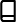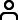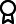Journal metrics
See full report
Acceptance rate17%
Submission to final decision78 days
Acceptance to publication44 days
CiteScore2.600
Journal Citation Indicator0.660
Impact Factor1.6

### Oscillation and Asymptotic Behavior of Three-Dimensional Third-Order Delay Systems

####Journal profile

International Journal of Differential Equations publishes research on differential equations, and related integral equations, from all scientists who use differential equations as tools within their own discipline.

####Editor spotlight

International Journal of Differential Equations maintains an Editorial Board of practicing researchers from around the world, to ensure manuscripts are handled by editors who are experts in the field of study.

####Special Issues

Do you think there is an emerging area of research that really needs to be highlighted? Or an existing research area that has been overlooked or would benefit from deeper investigation? Raise the profile of a research area by leading a Special Issue.

## Latest Articles

More articles
Research Article

### Solving the Fractional Schrödinger Equation with Singular Initial Data in the Extended Colombeau Algebra of Generalized Functions

This manuscript aims to highlight the existence and uniqueness results for the following Schrödinger problem in the extended Colombeau algebra of generalized functions. where is the Dirac distribution. The proofs of our main results are based on the Gronwall inequality and regularization method. We conclude our article by establishing the association concept of solutions.

Research Article

### On the Existence and Stability of Bounded Solutions for Abstract Dynamic Equations on Time Scales

In this article we study the existence and stability of bounded solutions for semilinear abstract dynamic equations on time scales in Banach spaces. In order to do so, we use the definition of the Riemann delta-integral to prove a result about closed operator in Banach spaces and then we just use the representation of bounded solutions as an improper delta-integral from minus infinite to . We prove the existence, uniqueness, and exponential stability of such bounded solutions. As particular cases, we study the existence of periodic and almost periodic solutions as well. Finally, we present some equations on time scales where our results can be applied.

Research Article

### Existence and Uniqueness of Renormalized Solution to Nonlinear Anisotropic Elliptic Problems with Variable Exponent and -Data

Nonlinear partial differential equations are considered as an essential tool for describing the behavior of many natural phenomena. The modeling of some phenomena requires to work in Sobolev spaces with constant exponent. But for others, such as electrorheological fluids, the properties of classical spaces are not sufficient to have precision. To overcome this difficulty, we work in the appropriate spaces called Lebesgue and Sobolev spaces with variable exponent. In recent works, researchers are attracted by the study of mathematical problems in the context of variable exponent. This great interest is motivated by their applications in many fields such as elastic mechanics, fluid dynamics, and image restoration. In this paper, we combine the technic of monotone operators in Banach spaces and approximation methods to prove the existence of renormalized solutions of a class of nonlinear anisotropic problem involving Leray–Lions operator, a graph, and data. In particular, we establish the uniqueness of the solution when the graph data are considered a strictly increasing function.

Research Article

### Bessel-Riesz Operators on Lebesgue Spaces and Morrey Spaces Defined in Measure Metric Spaces

The boundedness of Bessel–Riesz operators defined on Lebesgue spaces and Morrey spaces in measure metric spaces is discussed in this research study. The maximal operator and traditional dyadic decomposition are used to study the Bessel-Riesz operators. We investigate the interaction between the kernel and space parameters to get the results and see how this affects kernel-bound operators.

Research Article

### Solving Nonlinear Partial Differential Equations of Special Kinds of 3rd Order Using Balance Method and Its Models

Most nonlinear partial differential equations have many applications in the physical world. Finding solutions to nonlinear partial differential equations is not easily solvable and hence different modified techniques are applied to get solutions to such nonlinear partial differential equations. Among them, we considered the modified Korteweg–de Vries third order using the balance method and constructing its models using certain parameters. The method is successfully implemented in solving the stated equations. We obtained kind one and two soliton solutions and their graphical models are shown using mathematical software-12. The obtained results lead to shallow wave models. A few illustrative examples were presented to demonstrate the applicability of the models. Furthermore, physical and geometrical interpretations are considered for different parameters to investigate the nature of soliton solutions to their models. Finally, the proposed method is a standard, effective, and easily computable method for solving the modified Korteweg–de Vries equations and determining its perspective models.

Research Article

### A Fractional-Order Eco-Epidemiological Leslie–Gower Model with Double Allee Effect and Disease in Predator

In this paper, a fractional order of a modified Leslie–Gower predator-prey model with disease and the double Allee effect in predator population is proposed. Then, we analyze the important mathematical features of the proposed model such as the existence and uniqueness as well as the non-negativity and boundedness of solutions to the fractional-order system. Moreover, the local and global asymptotic stability conditions of all possible equilibrium points are investigated using Matignon’s condition and by constructing a suitable Lyapunov function, respectively. Finally, numerical simulations are presented to verify the theoretical results. We show numerically the occurrence of two limit cycles simultaneously driven by the order of the derivative, the bistability phenomenon for both the weak and strong Allee effect cases, and more dynamic behaviors such as the forward, backward, and saddle-node bifurcations which are driven by the transmission rate. We have found that the risk of extinction for the predator with a strong Allee effect is much higher when the spread of disease is relatively high.Journal metrics
See full report
Acceptance rate17%
Submission to final decision78 days
Acceptance to publication44 days
CiteScore2.600
Journal Citation Indicator0.660
Impact Factor1.6Author guidelinesEditorial boardDatabases and indexingAuthor guidelinesEditorial boardDatabases and indexing

Article of the Year Award: Outstanding research contributions of 2021, as selected by our Chief Editors. Read the winning articles.